# Resistance

Resistance is the measure of difficulty of passing an electric current through a material (measured in ohms).

If the material is rubber it has a very high resistance.

If the material is copper it has a very low resistance.

To visualise resistance scientists have found that a 250mm length of graphite pencil should have 1 unit of resistance (1 ohm)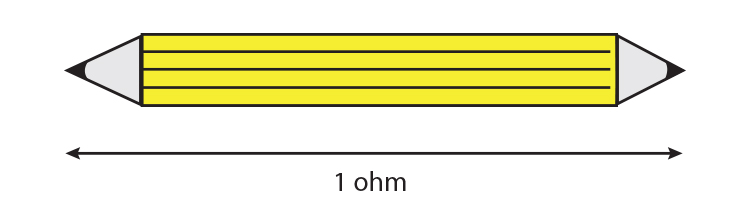NOTE: Don't forget to sharpen both ends

Another way of looking at resistance is to consider the following: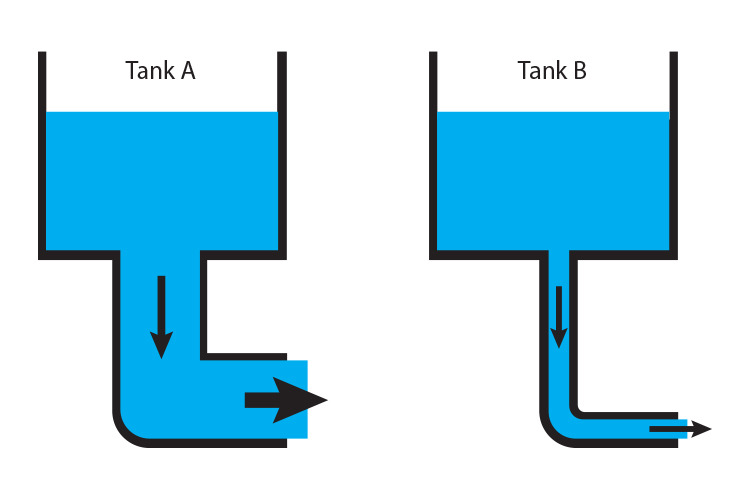There is less resistance in Tank A and it will empty a lot faster than Tank B.

So too in terms of pipes.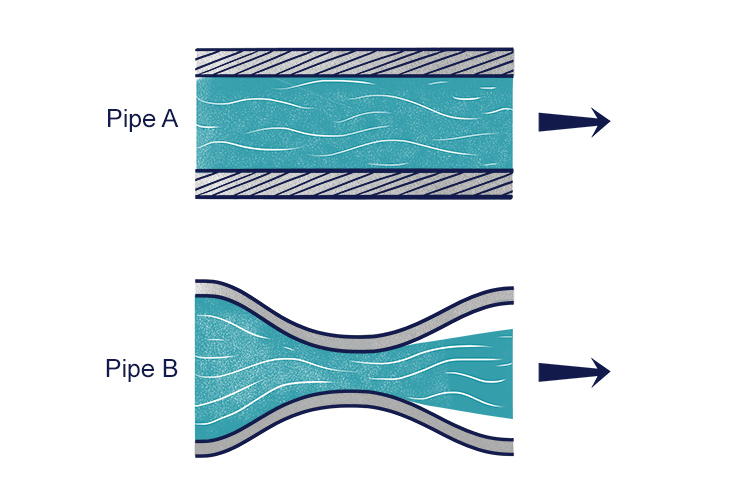There is less resistance in Pipe A and therefore flow will be a lot faster through it than Pipe B.

Also, there is less resistance in copper than rubber in electron flow: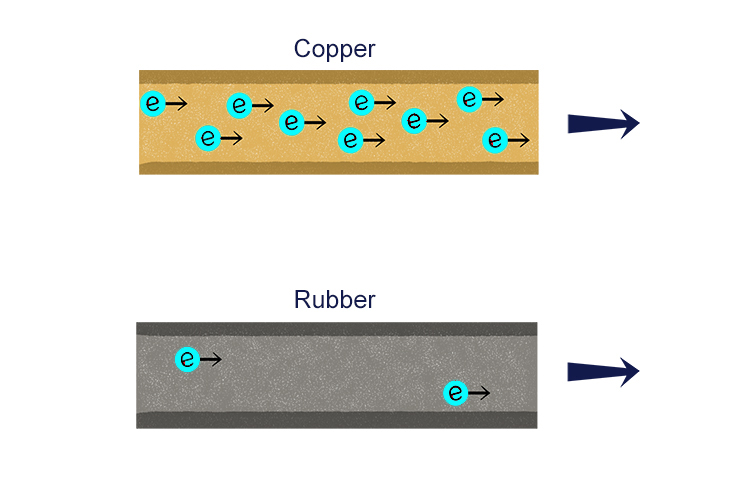Another way of thinking about resistance is hair getting stuck in a pipe.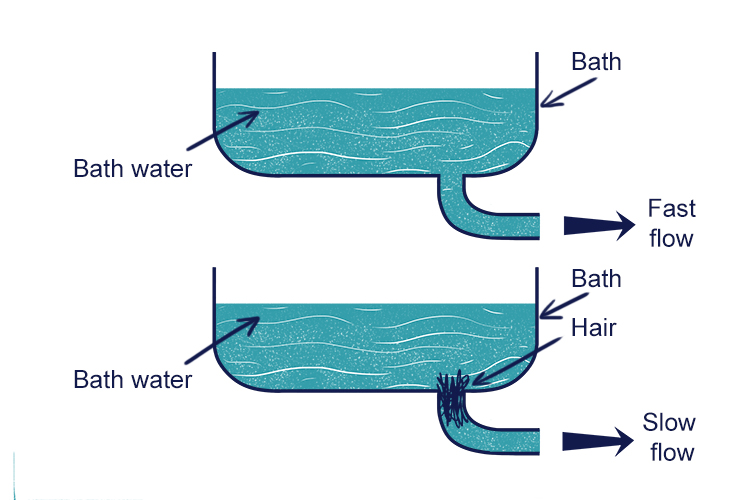Resistance = Hair